# Pitfalls and potential¶

## Introduction¶

This page shows how you can implement the examples provided in Chapter 2 of O’Sullivan and Unwin (2010). To get most out of this, go through the examples slowly, line by line. You should inspect the objects created and read the help files associated with the functions used.

## The Modifiable Areal Unit Problem¶

Below we recreate the data shown on page 37. There is one region that is divided into 6 x 6 = 36 grid cells. For each cell we have values for two variables. These gridded data can be represented as a matrix, but the easiest way to enter the values is to use a vector (which we can transform to a matrix later). I used line breaks for ease of comparison with the book such that it looks like a matrix anyway.

```# independent variable
ind <- c(87, 95, 72, 37, 44, 24,
40, 55, 55, 38, 88, 34,
41, 30, 26, 35, 38, 24,
14, 56, 37, 34,  8, 18,
49, 44, 51, 67, 17, 37,
55, 25, 33, 32, 59, 54)
# dependent variable
dep <- c(72, 75, 85, 29, 58, 30,
50, 60, 49, 46, 84, 23,
21, 46, 22, 42, 45, 14,
19, 36, 48, 23,  8, 29,
38, 47, 52, 52, 22, 48,
58, 40, 46, 38, 35, 55)
```

Now that we have the values, we can make a scatter plot.

```plot(ind, dep)
```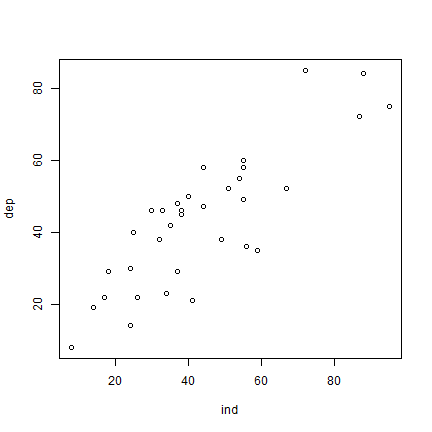And here is how you can fit a linear regression model using the `glm` function. `dep ~ ind` means ‘dep’ is a function of ‘ind’.

```m <- glm(dep ~ ind)
```

Now let’s look at our model `m`.

```m
##
## Call:  glm(formula = dep ~ ind)
##
## Coefficients:
## (Intercept)          ind
##     10.3750       0.7543
##
## Degrees of Freedom: 35 Total (i.e. Null);  34 Residual
## Null Deviance:       12080
## Residual Deviance: 3742  AIC: 275.3
```

To get a bit more information about `m`, we can use the `summary` function.

```s <- summary(m)
s
##
## Call:
## glm(formula = dep ~ ind)
##
## Coefficients:
##             Estimate Std. Error t value Pr(>|t|)
## (Intercept) 10.37497    4.12773   2.513   0.0169 *
## ind          0.75435    0.08668   8.703 3.61e-10 ***
## ---
## Signif. codes:  0 '***' 0.001 '**' 0.01 '*' 0.05 '.' 0.1 ' ' 1
##
## (Dispersion parameter for gaussian family taken to be 110.0631)
##
##     Null deviance: 12078.8  on 35  degrees of freedom
## Residual deviance:  3742.1  on 34  degrees of freedom
## AIC: 275.34
##
## Number of Fisher Scoring iterations: 2
```

We can use `m` to add a regression line to our scatter plot.

```plot(ind, dep)
abline(m)
```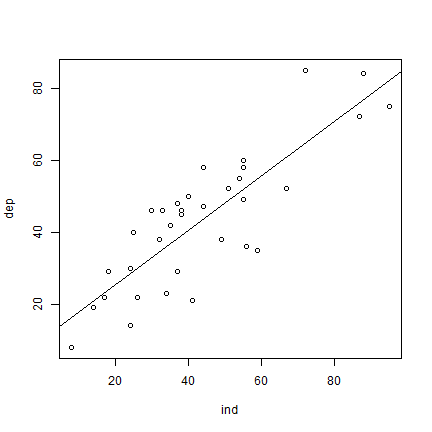OK. But let’s see how to make a plot that looks more like the one in the book. I first set up a plot without axes, and then add the two axes I want (in stead of the standard box). `las=1` rotates the labels to a horizontal position. The arguments `yaxs="i"`, and `xaxs="i"` force the axes to be drawn at the edges of the plot window (overwriting the default to enlarge the ranges by 6%). To get the filled diamond symbol, I use `pch=18`. See `plot(1:25, pch=1:25)` for more numbered symbols.

Then I add the formula by extracting the coefficients from the regression summary object `s` that was created above, and by concatenating the text elements with the `paste0` function. Creating the superscript in R2 also takes some fiddling. Don’t worry about understanding the details of that. There are a few alternative ways to do this, all of them can be found on-line, so there is no need to remember how to do it.

The regression line should only cover the range (min to max value) of variable `ind`. An easy way to do that is to use the regression model to predict values for these extremes and draw a line between these.

```plot(ind, dep, pch=18, xlim=c(0,100), ylim=c(0,100),
axes=FALSE, xlab='', ylab='', yaxs="i", xaxs="i")
axis(1, at=(0:5)*20)
axis(2, at=(0:5)*20, las=1)
# create regression formula
f <- paste0('y = ', round(s\$coefficients, 4), 'x + ', round(s\$coefficients, 4))
# add the text in variable f to the plot
text(0, 96, f, pos=4)
# compute r-squared
R2 <- cor(dep, predict(m))^2
# set up the expression (a bit complex, this)
r2 <- bquote(italic(R)^2 == .(round(R2, 4)))
# and add it to the plot
text(0, 85, r2, pos=4)
# compute regression line
# create a data.frame with the range (minimum and maximum) of values of ind
px <- data.frame(ind = range(ind))
# use the regression model to get predicted value for dep at these two extremes
py <- predict(m, px)
# combine the min and max values and the predicted values
ln <- cbind(px, py)
# add to the plot as a line
lines(ln, lwd=2)
```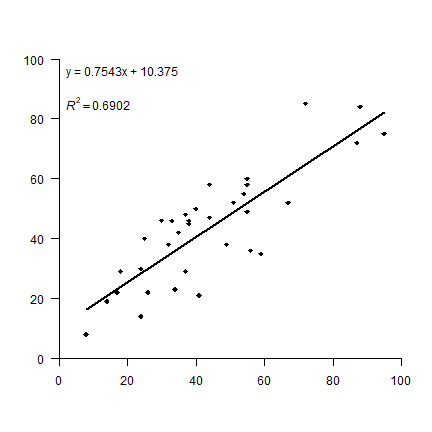Now the aggregation. I first turn the vectors into matrices, which is very easy to do. You should play with the matrix function a bit to see how it works. It is particularly important that you understand the argument `byrow=TRUE`. By default `R` fills matrices column-wise.

```mi <- matrix(ind, ncol=6, nrow=6, byrow=TRUE)
md <- matrix(dep, ncol=6, nrow=6, byrow=TRUE)
```

Question 1: Create these matrices from `ind` and `dep` without using `byrow=TRUE`. Hint: use the `t` function after you made the matrix.

The type of aggregation as shown in Figure 2.1 is not a very typical operation in the context of matrix manipulation. However, it is very common to do this with raster data. So let’s first transform the matrices to objects that represent raster data, `SpatRaster` objects in this case. This class is defined in the `terra` package, so we need to load that first. If `library(terra)` gives this error: `Error in library("terra") : there is no package called ‘terra’` you need to install the package first, using this command: `install.packages("terra")`.

```# load package
library(terra)
## terra 1.7.62
# turn matrices into SpatRaster objects
ri <- rast(mi)
rd <- rast(md)
```

Inspect one of these new objects

```ri
## class       : SpatRaster
## dimensions  : 6, 6, 1  (nrow, ncol, nlyr)
## resolution  : 1, 1  (x, y)
## extent      : 0, 6, 0, 6  (xmin, xmax, ymin, ymax)
## coord. ref. :
## source(s)   : memory
## name        : lyr.1
## min value   :     8
## max value   :    95
plot(ri, legend=FALSE)
text(ri)
```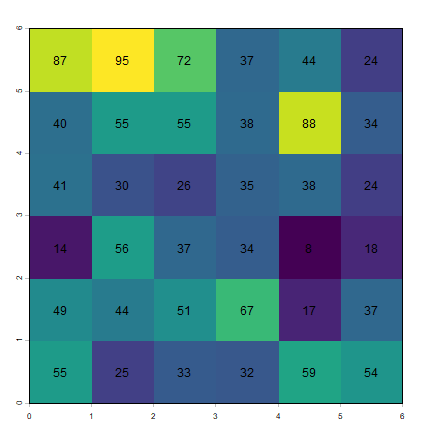The `raster` package has an aggregate function that we will use. We specify that we want to aggregate sets of 2 columns, but not aggregate rows. The values for the new cells should be computed from the original cells using the mean function.

Question 2: Instead of the ``mean`` function What other functions could, in principle, reasonably be used in an aggregation of raster cells?

```ai1 <- aggregate(ri, c(2, 1), fun=mean)
ad1 <- aggregate(rd, c(2, 1), fun=mean)
```

Inspect the results

```as.matrix(ai1)
##       lyr.1
##  [1,]  63.5
##  [2,]  75.0
##  [3,]  63.5
##  [4,]  37.5
##  [5,]  66.0
##  [6,]  29.0
##  [7,]  27.5
##  [8,]  43.0
##  [9,]  31.5
## [10,]  34.5
## [11,]  23.0
## [12,]  21.0
## [13,]  52.0
## [14,]  34.5
## [15,]  42.0
## [16,]  49.5
## [17,]  38.0
## [18,]  45.5
plot(ai1)
text(ai1, digits=1)
```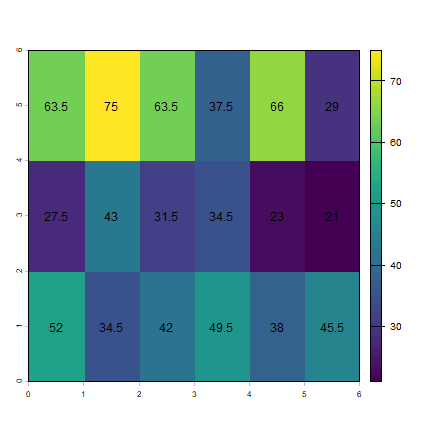To be able to do the regression as we did above, I first combine the two `SpatRaster` objects into a (multi-layer) object.

```s1 <- c(ai1, ad1)
names(s1) <- c("ind", "dep")
s1
## class       : SpatRaster
## dimensions  : 3, 6, 2  (nrow, ncol, nlyr)
## resolution  : 1, 2  (x, y)
## extent      : 0, 6, 0, 6  (xmin, xmax, ymin, ymax)
## coord. ref. :
## source(s)   : memory
## names       : ind, dep
## min values  :  21,  20
## max values  :  75,  71
plot(s1)
```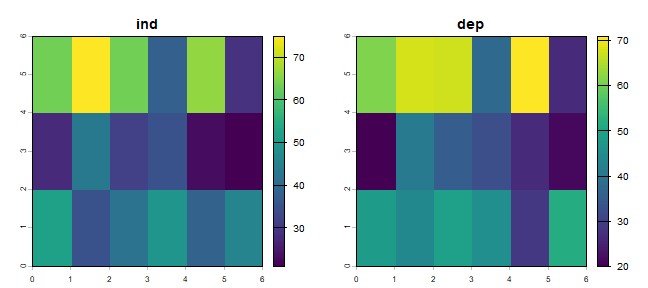Below I coerce the `SpatRaster` into a `data.frame`. In `R`, most functions for statistical analysis want the input data as a `data.frame`.

```d1 <- as.data.frame(s1)
##    ind  dep
## 1 63.5 61.0
## 2 75.0 67.5
## 3 63.5 67.0
## 4 37.5 37.5
## 5 66.0 71.0
## 6 29.0 26.5
```

To recap: each matrix was used to create a `SpatRaster` that we aggregated and then combined. Each of the aggregated `SpatRaster` layers became a single variable (column) in the `data.frame`. If would perhaps have been more efficient to first make a `SpatRaster` and then `aggregate`.

Question 3: There are other ways to do the above (converting two `SpatRaster` objects to a `data.frame`). Show how to obtain the same result (`d1`) using `as.vector` and `cbind`.

Let’s fit a regression model again, now with these aggregated data:

```ma1 <- glm(dep~ind, data=d1)
```

Same idea for for the other aggregation (‘Aggregation scheme 2’). But note that the arguments to the `aggregate` function are, of course, different.

```ai2 <- aggregate(ri, c(1, 2), fun=mean)
ad2 <- aggregate(rd, c(1, 2), fun=mean)
plot(ai2)
text(ai2, digits=1, srt=90)
```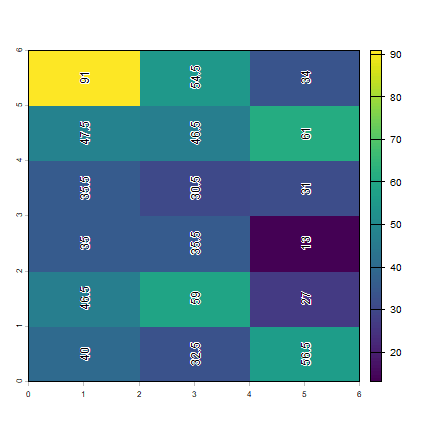```s2 <- c(ai2, ad2)
names(s2) <- c('ind', 'dep')
# coerce to data.frame
d2 <- as.data.frame(s2)
ma2 <- glm(dep ~ ind, data=d2)
```

Now we have three regression model objects. We first created object `m`, and then the two models with aggregated data: `ma1` and `ma2`. Compare the regression model coefficients.

```m\$coefficients
## (Intercept)         ind
##  10.3749675   0.7543472
ma1\$coefficients
## (Intercept)         ind
##   1.2570386   0.9657093
ma2\$coefficients
## (Intercept)         ind
##  13.5899183   0.6798216
```

Re-creating figure 2.1 takes some effort. We want to make a similar figure three times (two matrices and a plot). That makes it efficient and practical to use a function. Look here if you do not remember how to write and use your own function in `R`:

The function I wrote, called `plotMAUP`, is a bit complex, so I do not show it here. But you can find it in the source code for this page. Have a look at it if you can, don’t worry about the details, but see if you can understand the main reason for each step. It helps to try the lines of the function one by one (outside of the function).

To use the `plotMAUP` function, I first set up a plotting canvas of 3 rows and 3 columns, using the `mfrow` argument in the `par` function. The `par` function is very important for customizing plots — and it has an overwhelming number of options to consider. See `?par`. The `mai` argument is used to change the margins around each plot.

```# plotting parameters
par(mfrow=c(3,3), mai=c(0.25,0.15,0.25,0.15))
# Now call plotMAUP 3 times
plotMAUP(ri, rd, title=c('Independent variable', 'Dependent variable'))
# aggregation scheme 1
# aggregation scheme 2
```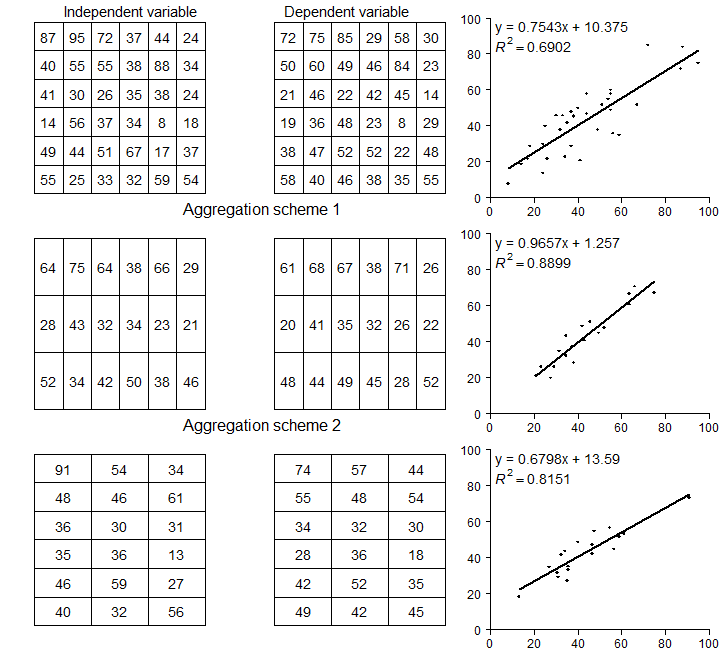Figure 2.1 An illustration of MAUP

Here we explore the data in Figure 2.2 (page 46). The values used are not exactly the same (as they were not provided in the text), but it is all very similar.

Set up the data, using x-y coordinates for each point:

```A <- c(40, 43)
B <- c(1, 101)
C <- c(54, 111)
D <- c(104, 65)
E <- c(60, 22)
F <- c(20, 2)
pts <- rbind(A,B,C,D,E,F)
##   [,1] [,2]
## A   40   43
## B    1  101
## C   54  111
## D  104   65
## E   60   22
## F   20    2
```

Plot the points and labels:

```plot(pts, xlim=c(0,120), ylim=c(0,120), pch=20, cex=2, col='red', xlab='X', ylab='Y', las=1)
text(pts+5, LETTERS[1:6])
```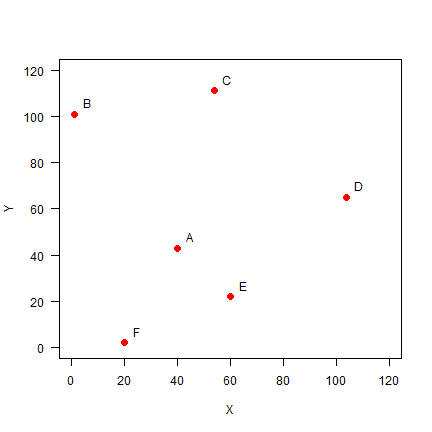### Distance¶

It is easy to make a distance matrix (see page 47)

```dis <- dist(pts)
dis
##           A         B         C         D         E
## B  69.89278
## C  69.42622  53.93515
## D  67.67570 109.11004  67.94115
## E  29.00000  98.60020  89.20202  61.52235
## F  45.61798 100.80675 114.17968 105.00000  44.72136
D <- as.matrix(dis)
round(D)
##    A   B   C   D  E   F
## A  0  70  69  68 29  46
## B 70   0  54 109 99 101
## C 69  54   0  68 89 114
## D 68 109  68   0 62 105
## E 29  99  89  62  0  45
## F 46 101 114 105 45   0
```

Distance matrices are used in all kinds of non-geographical applications. For example, they are often used to create cluster diagrams (dendograms).

Question 4: Show R code to make a cluster dendogram summarizing the distances between these six sites, and plot it. See `?hclust`.

To get the adjacency matrix, here defined as points within a distance of 50 from each other is trivial given that we have the distances `D`.

```a <-  D < 50
a
##       A     B     C     D     E     F
## A  TRUE FALSE FALSE FALSE  TRUE  TRUE
## B FALSE  TRUE FALSE FALSE FALSE FALSE
## C FALSE FALSE  TRUE FALSE FALSE FALSE
## D FALSE FALSE FALSE  TRUE FALSE FALSE
## E  TRUE FALSE FALSE FALSE  TRUE  TRUE
## F  TRUE FALSE FALSE FALSE  TRUE  TRUE
```

To make this match matrix 2.6 on page 48, set the diagonal values to `NA` (we do not consider a point to be adjacent to itself). Also change the change the `TRUE/FALSE` values to to `1/0` using a simple trick (multiplication with 1)

```diag(a) <- NA
##    A  B  C  D  E  F
## A NA  0  0  0  1  1
## B  0 NA  0  0  0  0
## C  0  0 NA  0  0  0
## D  0  0  0 NA  0  0
## E  1  0  0  0 NA  1
## F  1  0  0  0  1 NA
```

#### Three nearest neighbors¶

Computing the “three nearest neighbors” adjacency-matrix requires a bit more advanced understanding of `R`.

For each row, we first get the column numbers in order of the values in that row (that is, the numbers indicate how the values are ordered).

```cols <- apply(D, 1, order)
# we need to transpose the result
cols <- t(cols)
```

And then get columns 2 to 4 (why not column 1?)

```cols <- cols[, 2:4]
cols
##   [,1] [,2] [,3]
## A    5    6    4
## B    3    1    5
## C    2    4    1
## D    5    1    3
## E    1    6    4
## F    5    1    2
```

As we now have the column numbers, we can make the row-column pairs that we want (`rowcols`).

```rowcols <- cbind(rep(1:6, each=3), as.vector(t(cols)))
##      [,1] [,2]
## [1,]    1    5
## [2,]    1    6
## [3,]    1    4
## [4,]    2    3
## [5,]    2    1
## [6,]    2    5
```

We use these pairs as indices to change the values in matrix `Ak3`.

```Ak3 <- adj50 * 0
Ak3[rowcols] <- 1
Ak3
##    A  B  C  D  E  F
## A NA  0  0  1  1  1
## B  1 NA  1  0  1  0
## C  1  1 NA  1  0  0
## D  1  0  1 NA  1  0
## E  1  0  0  1 NA  1
## F  1  1  0  0  1 NA
```

#### Weights matrix¶

Getting the weights matrix is simple.

```W <- 1 / D
round(W, 4)
##        A      B      C      D      E      F
## A    Inf 0.0143 0.0144 0.0148 0.0345 0.0219
## B 0.0143    Inf 0.0185 0.0092 0.0101 0.0099
## C 0.0144 0.0185    Inf 0.0147 0.0112 0.0088
## D 0.0148 0.0092 0.0147    Inf 0.0163 0.0095
## E 0.0345 0.0101 0.0112 0.0163    Inf 0.0224
## F 0.0219 0.0099 0.0088 0.0095 0.0224    Inf
```

Row-normalization is not that difficult either. First get rid if the `Inf` values by changing them to `NA`. (Where did the `Inf` values come from?)

```W[!is.finite(W)] <- NA
```

Then compute the row sums.

```rtot <- rowSums(W, na.rm=TRUE)
# this is equivalent to
# rtot <- apply(W, 1, sum, na.rm=TRUE)
rtot
##          A          B          C          D          E          F
## 0.09989170 0.06207541 0.06763182 0.06443810 0.09445017 0.07248377
```

Divide the rows by their totals and check if they row sums add up to 1.

```W <- W / rtot
rowSums(W, na.rm=TRUE)
## A B C D E F
## 1 1 1 1 1 1
```

The values in the columns do not add up to 1.

```colSums(W, na.rm=TRUE)
##         A         B         C         D         E         F
## 1.3402904 0.8038417 0.9108116 0.8166821 1.2350790 0.8932953
```

Question 5: Show how you can do ‘column-normalization’ (Just an exercise, in spatial data analysis this is not a typical thing to do).

### Proximity polygons¶

Proximity polygons are discussed on pages 50-52. Here I show how you can compute these with the `voronoi` function. We use the data from the previous example.

```v <- voronoi(vect(pts))
```

Here is a plot of our proximity polygons (also known as a Voronoi diagram).

```par(mai=rep(0,4))
plot(v, lwd=4, border='gray', col=rainbow(6))
points(pts, pch=20, cex=2)
text(pts+5, toupper(letters[1:6]), cex=1.5)
```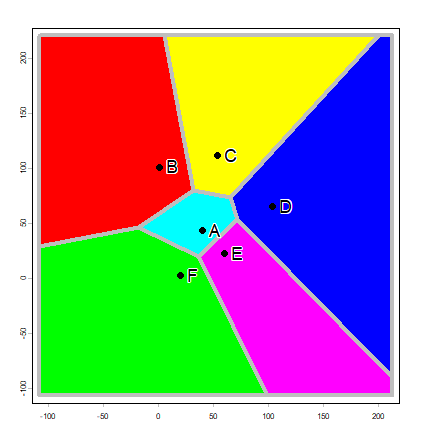Note that the `voronoi` functions returns a `SpatVector`. This is a class (type of object) that can be used to represent geospatial polygons in R.

```class(v)
##  "SpatVector"
## attr(,"package")
##  "terra"
v
##  class       : SpatVector
##  geometry    : polygons
##  dimensions  : 6, 0  (geometries, attributes)
##  extent      : -108, 213, -107, 220  (xmin, xmax, ymin, ymax)
##  coord. ref. :
```